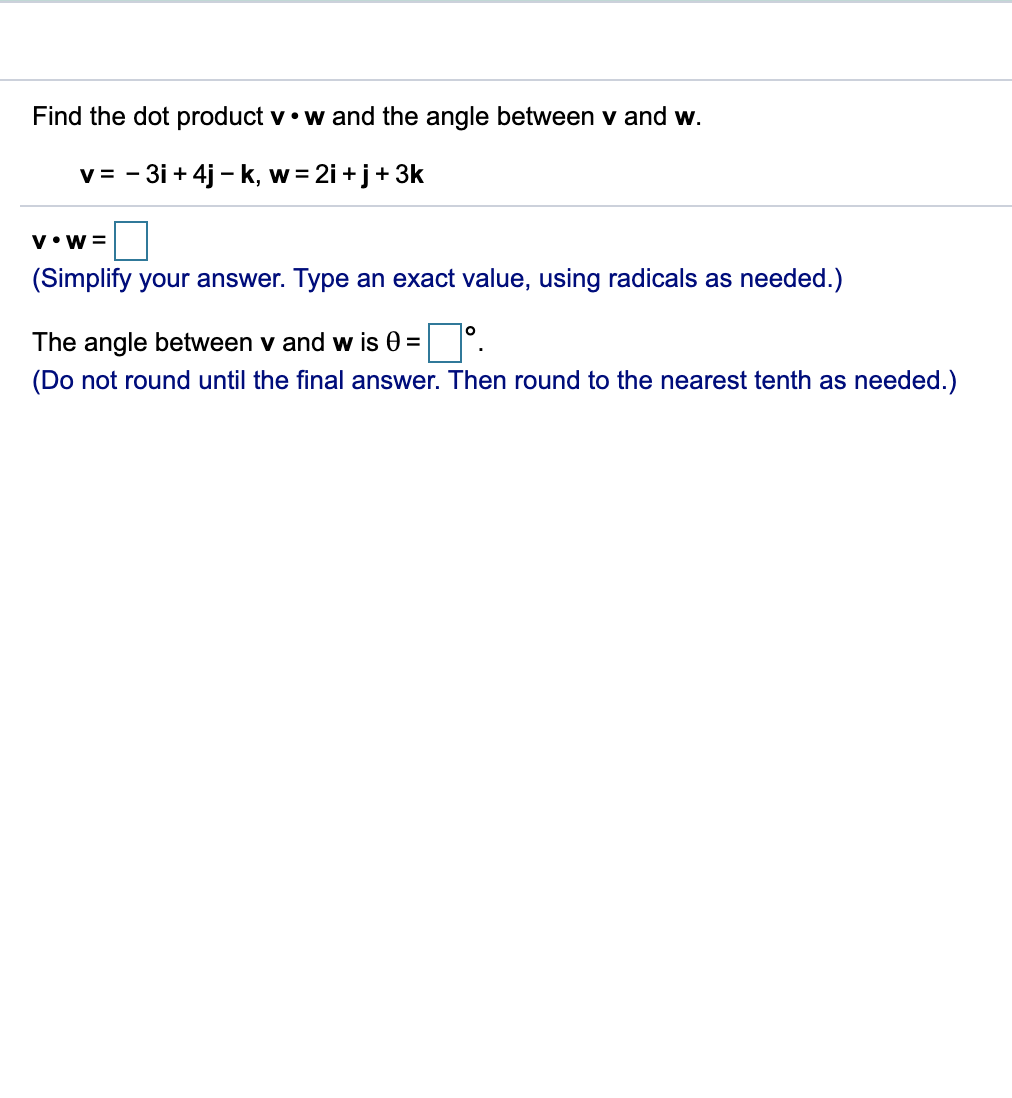# Find the dot productv.w and the angle between v and w.v-3i+4j k, w2i +j 3kW =V W(Simplify your answer. Type an exact value, using radicals as needed.)OThe angle between v and w is 0 =(Do not round until the final answer. Then round to the nearest tenth as needed.)

Question
3 viewshelp_outlineImage TranscriptioncloseFind the dot product v.w and the angle between v and w. v -3i+4j k, w 2i +j 3k W = V W (Simplify your answer. Type an exact value, using radicals as needed.) O The angle between v and w is 0 = (Do not round until the final answer. Then round to the nearest tenth as needed.) fullscreen
check_circle

Step 1

Firstly,  we find vectors v and w .

Step 2

Then we find the cosine of angle between the vectors

Step 3

Now, we find angle betw...

### Want to see the full answer?

See Solution

#### Want to see this answer and more?

Solutions are written by subject experts who are available 24/7. Questions are typically answered within 1 hour.*

See Solution
*Response times may vary by subject and question.
Tagged in

### Other